Jeopardy Final Jeopardy Types of Activity reactions I

• Slides: 28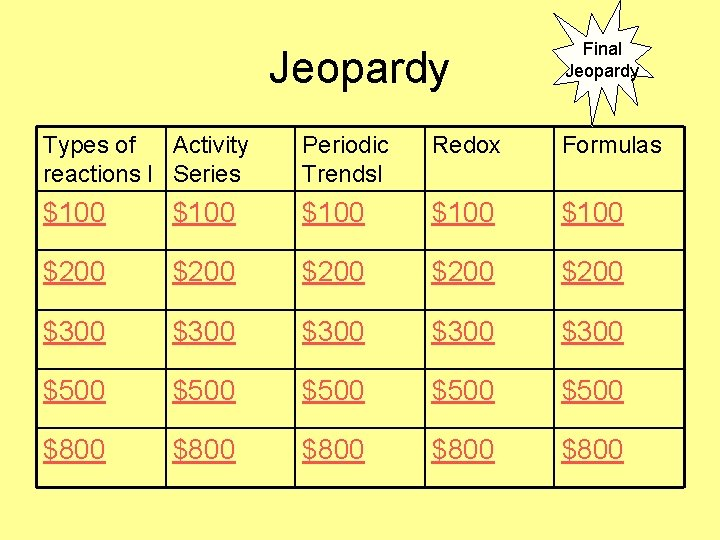Jeopardy Final Jeopardy Types of Activity reactions I Series Periodic Trends. I Redox Formulas \$100 \$100 \$200 \$200 \$300 \$300 \$500 \$500 \$800 \$800\$100 Types of reactions • This type of reaction occurs when Mg burns in oxygen gas to produce magnesium oxide.\$200 Types of reactions • In this type of reaction 2 H 2 O 2 H 2 + O 2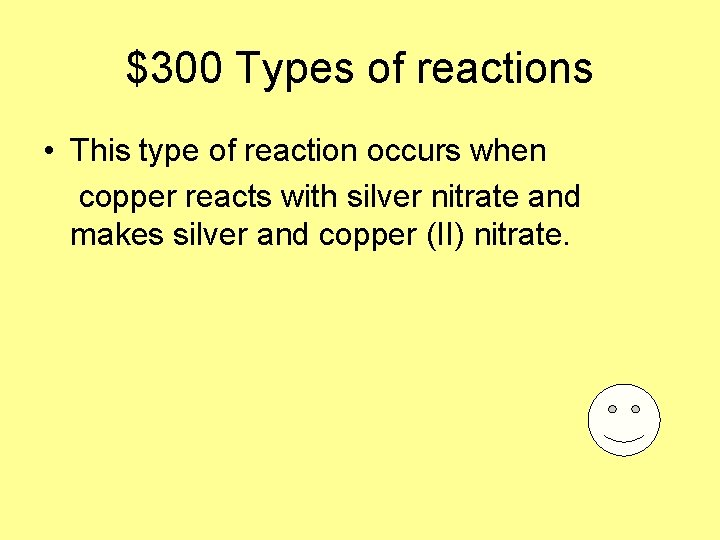\$300 Types of reactions • This type of reaction occurs when copper reacts with silver nitrate and makes silver and copper (II) nitrate.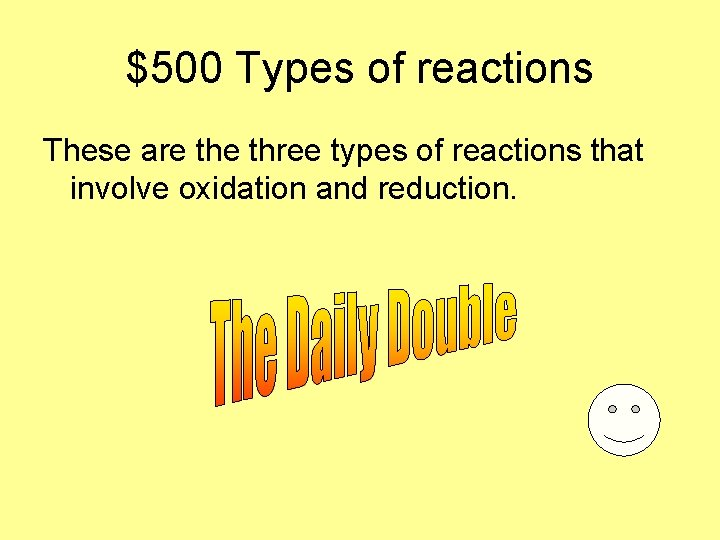\$500 Types of reactions These are three types of reactions that involve oxidation and reduction.\$800 Types of reactions • This is the type of reaction that occurs when sodium is put in water and hydrogen gas forms. • 2 Na + 2 HOH H 2 + 2 Na. OH\$100 Activity Series • This is the rule for deciding what will happen in a reaction using the activity series.\$200 Activity Series • This nonmetal element is above silver in the activity series. • • • Lithium Potassium Calcium Sodium Magnesium Aluminum Zinc Iron Lead Hydrogen Copper Silver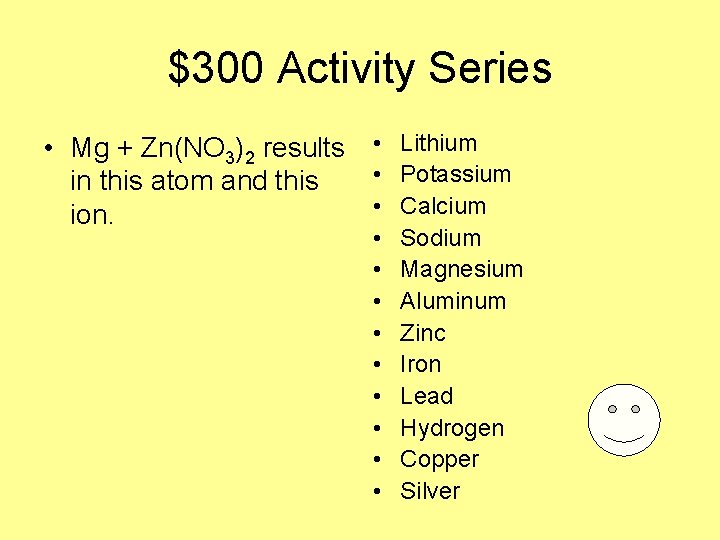\$300 Activity Series • Mg + Zn(NO 3)2 results • Lithium • Potassium in this atom and this • Calcium ion. • • • Sodium Magnesium Aluminum Zinc Iron Lead Hydrogen Copper Silver\$500 Activity Series • Zinc metal is added to HCl, hydrochloric acid, and this gas is the product. • • • Lithium Potassium Calcium Sodium Magnesium Aluminum Zinc Iron Lead Hydrogen Copper Silver\$800 Activity Series • These two precious metals are below silver in the activity series. • • • Lithium Potassium Calcium Sodium Magnesium Aluminum Zinc Iron Lead Hydrogen Copper Silver\$100 Periodic Trends • In family I, among Na, Li, K, and Rb, this atom has the largest radius.\$200 Periodic Trends • This is the ionization energy equation for making a cation from potassium. K + ? K+ + ? The ? represent the missing terms.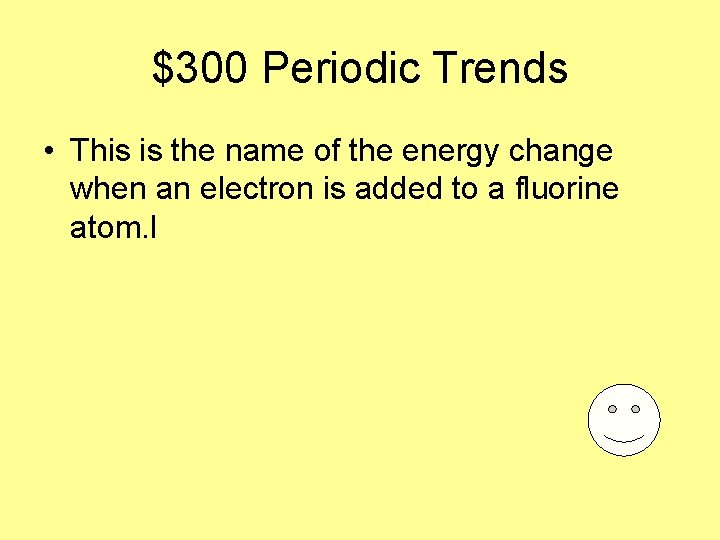\$300 Periodic Trends • This is the name of the energy change when an electron is added to a fluorine atom. l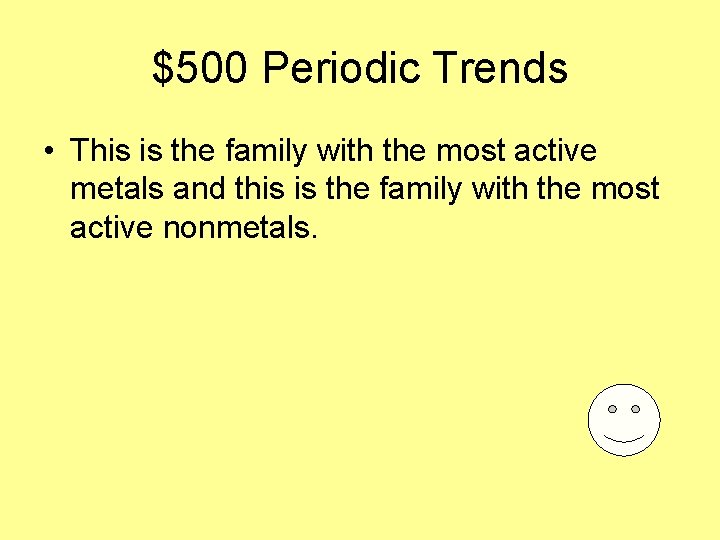\$500 Periodic Trends • This is the family with the most active metals and this is the family with the most active nonmetals.\$800 Periodic Trends • As the atomic number increases in a period, these are the increases and decreases in each: radius ionization energy electron affinity energy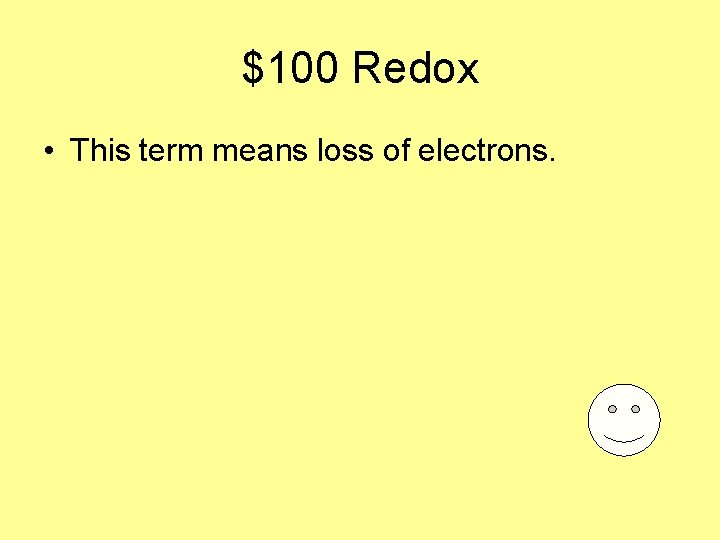\$100 Redox • This term means loss of electrons.\$200 Redox • This term means gain of electrons and causes an ion to have less positive charge.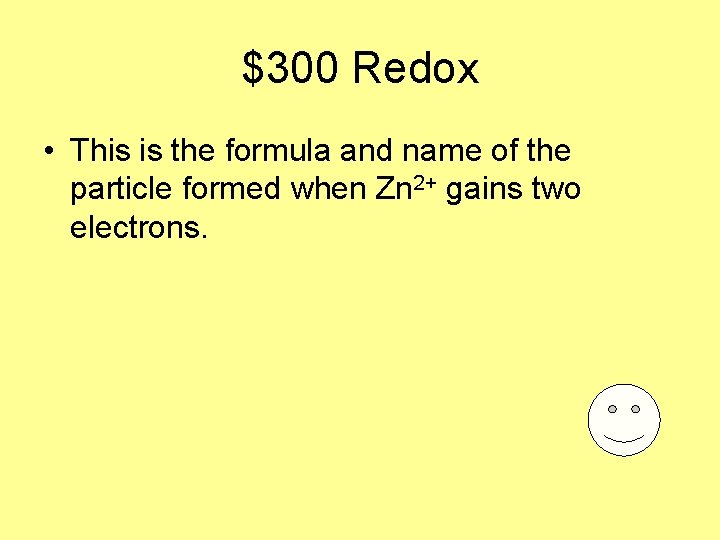\$300 Redox • This is the formula and name of the particle formed when Zn 2+ gains two electrons.\$500 Redox • These are the products of the reaction: Mg: + Cu 2+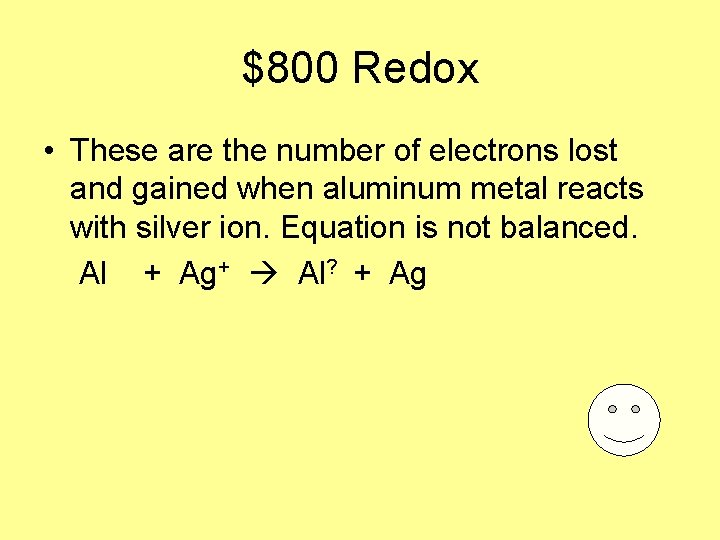\$800 Redox • These are the number of electrons lost and gained when aluminum metal reacts with silver ion. Equation is not balanced. Al + Ag+ Al? + Ag\$100 Formulas • This is the formula of sodium chloride.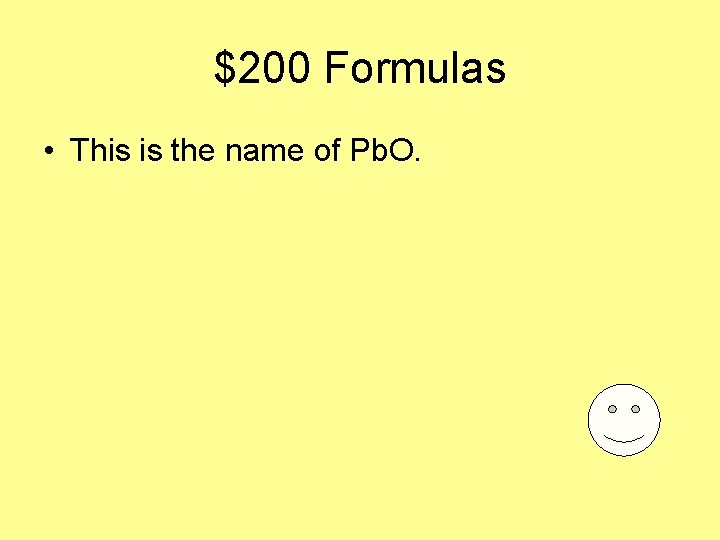\$200 Formulas • This is the name of Pb. O.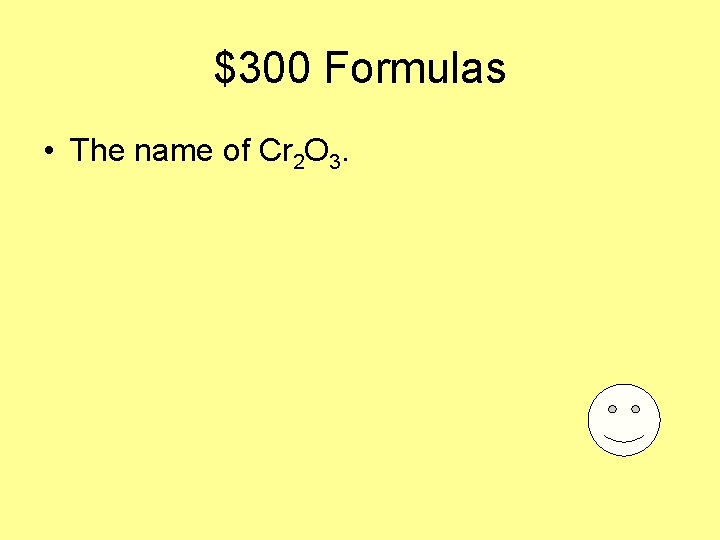\$300 Formulas • The name of Cr 2 O 3.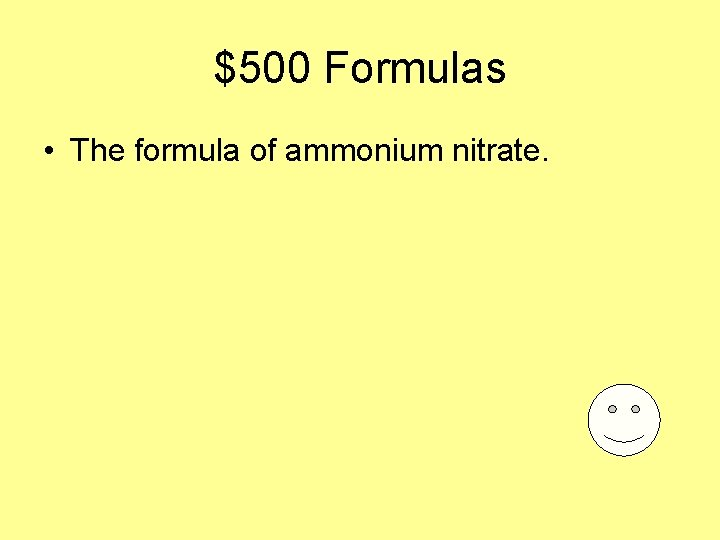\$500 Formulas • The formula of ammonium nitrate.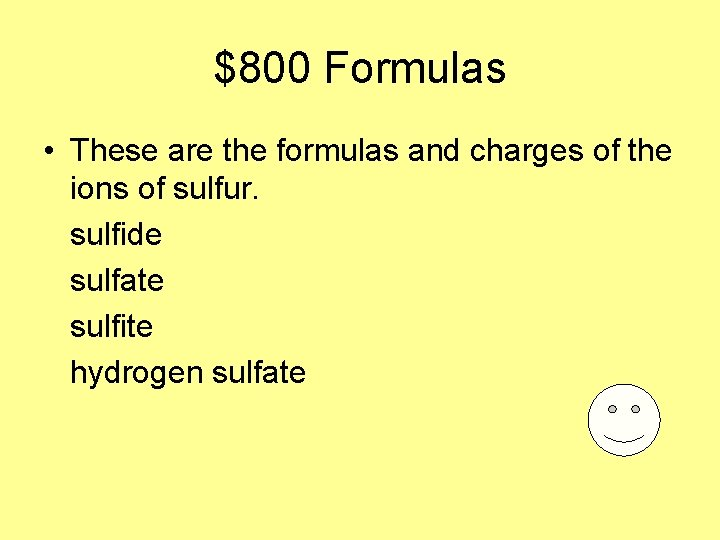\$800 Formulas • These are the formulas and charges of the ions of sulfur. sulfide sulfate sulfite hydrogen sulfate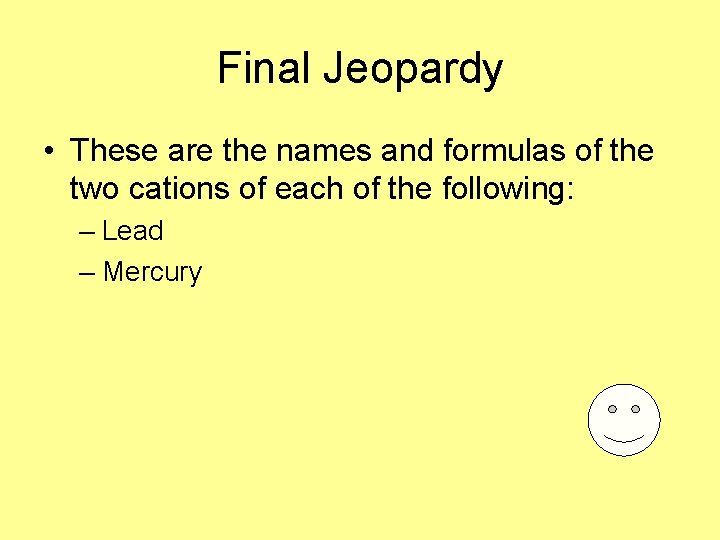Final Jeopardy • These are the names and formulas of the two cations of each of the following: – Lead – Mercury